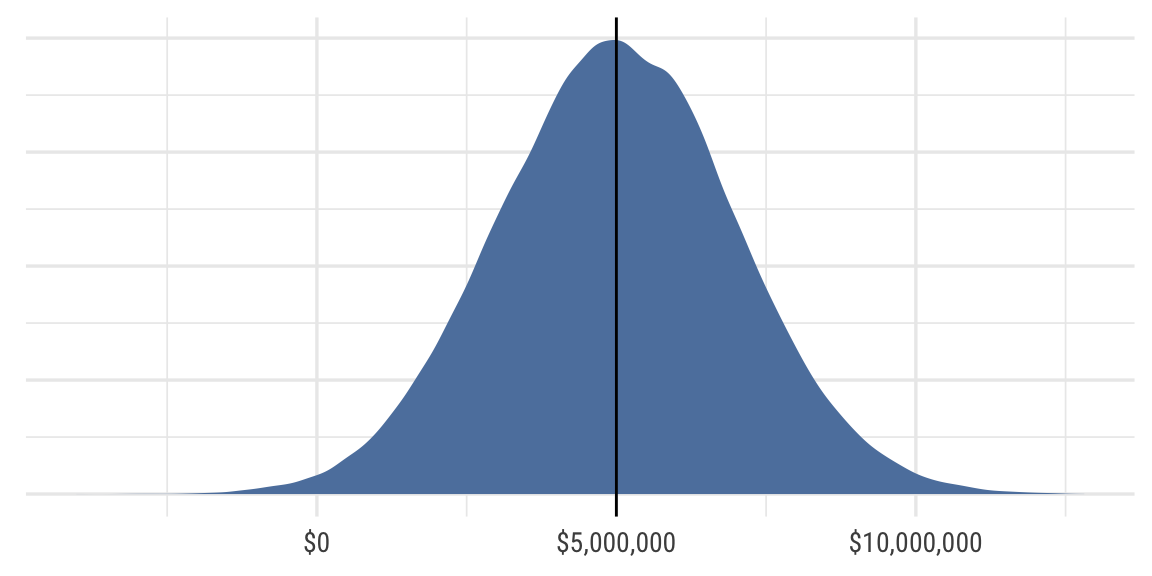# Evaluating policies I

Materials for class on Monday, April 8, 2019

## Present value

$PV = \frac{FV}{(1 + r)^t}$

## Cost benefit analysis playground

Consider this example:

• UDOT is considering building a new freeway that is estimated to save 100,000 hours of driving and 5 lives each year.
• The project will require 1 million bags of asphalt ($100/bag) and 1 million hours of labor (half at$20/hour, half at $10/hour). The construction occurs in year 0. • Starting in year 1, the freeway will require maintenance that will cost$18 million/year.
• Assume the following:
• Drivers estimate the value of their time at $17/hour • The value of a statistical life is$5 million
• The costs and benefits of the project will last for 25 years
• The discount rate is 4%

Answer these questions:

1. What is the NPV of this project? What is the benefit-cost ratio?

2. Use an Excel data table and a graph to show the NPV across a range of the following values. At what point does the project break even?
1. Discount rate
2. Hours of driving saved
3. Value of hour
4. Lives saved
3. The VSL is a statistical estimate of the amount people would be willing to pay to reduce their risk of death by 1%, extrapolated out to 100%.

Because VSL is an average, it has measures of uncertainty associated with it. According to academic research, there is substantial uncertainty in VSL estimates: in 2008, the NHTSA reported that the 95% confidence interval for VSL ranges from $1,000,000 to$10,000,000, which represents a standard deviation of around $2,000,000. With a$5 million average, the distribution would look something like this:Use Monte Carlo simulation in Excel to estimate the NPV over 1,000 trials, varying the VSL with a mean of $5 million and a standard deviation of$2 million. What is the average NPV over all these trials? What is the probability that the NPV is greater than \$0? Is this still a good project, given the uncertainty of the VSL?

## Clearest and muddiest things

Go to this form and answer these three questions:

1. What was the muddiest thing from class today? What are you still wondering about?
2. What was the clearest thing from class today?
3. What was the most exciting thing you learned?

I’ll compile the questions and send out answers after class.